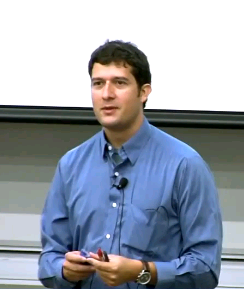# Math 2A: Calculus I (English)

## Course Information

UCI Math 2A is the first quarter in Single-Variable Calculus and covers the following topics: Introduction to derivatives, calculation of derivatives of algebraic and trigonometric functions; applications including curve sketching, related rates, and optimization. Exponential and logarithm functions.

Mathematics Dept. | Physical Sciences Sch. | University of California, Irvine
Keywords: calculus,single-variable,functions,inverse functions,derivatives,chain rule,compound interest,Mean Value Theorem,L'Hospital's Rule,antiderivatives,limits of infinityAuthor:
German Andres Enciso Ruiz
Title:
Assistant Professor
Department:
Mathematics